### Home > CALC > Chapter 4 > Lesson 4.1.2 > Problem4-24

4-24.
1. Determine the following limits quickly. Homework Help ✎

1.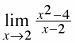2.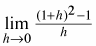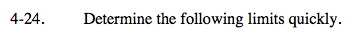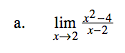Factor first. The denominator might 'cancel out.'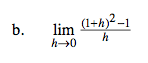This is Hana's definition of a derivative at a point.

$f'(a)=\lim_{h\rightarrow 0}\frac{f(a+h)-f(a)}{h}$

What is f(x)?
What is a?
What is f '(x)?
What is f '(a)?

f(x) = x2
a = 1
f '(x) = 2x
f '(a) = 2
Therefore the limit as h→0 = f '(a) = 2